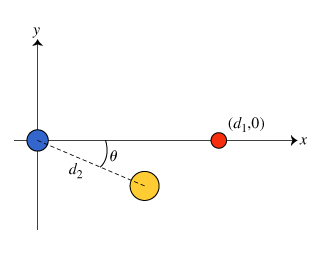# Question Solved1 Answer Consider the following configuration of fixed, uniformly charged spheres in (Figure 1) : a blue sphere fixed at the origin with positive charge q, a red sphere fixed at the point (d1,0) with unknown charge qred, and a yellow sphere fixed at the point (d2cos(θ),−d2sin(θ)) with unknown charge qyellow. The net electric force on the blue sphere has a (d1,00Consider the following configuration of fixed, uniformly charged spheres in (Figure 1) :

a blue sphere fixed at the origin with positive charge q,

a red sphere fixed at the point (d1,0) with unknown charge qred, and

a yellow sphere fixed at the point (d2cos(θ),−d2sin(θ)) with unknown charge qyellow.

The net electric force on the blue sphere has a magnitude F and is directed in the − y direction.

A.Suppose that the magnitude of the charge on the yellow sphere is determined to be 2q. Calculate the charge qred on the red sphere.

Transcribed Image Text: (d1,00
More
Transcribed Image Text: (d1,00